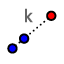# Dilation Exploration

Topic:
Dilation

## DIRECTIONS:

1) Select the slider tool to create a slider. Name the slider k. Set Min = -5, Max = 5, Increment = 0.1 2) Select the DILATE FROM POINTtool. Highlight a box around point A, point B, and Curious George's picture. Then select point C (to serve as center of dilation) In the pop-up box that appears, enter "k" (without the " " 's) to serve as the scale factor. 3) Select the Move tool. Explore! Please answer the questions that appear below the applet.

## 1)

Suppose point A = (1, 2) is dilated about C(0,0) with scale factor 2. Suppose point B = (4, 1) is dilated about C(0,0) with scale factor 2. What would the coordinates of the A' = image of A be? What would the coordinates of B' = image of B be?

## 2)

Suppose point A = (1, 2) is dilated about C(0,0) with scale factor 3. Suppose point B = (4, 1) is dilated about C(0,0) with scale factor 3. What would the coordinates of the A' = image of A be? What would the coordinates of B' = image of B be?

## 3)

Suppose point A = (1, 2) is dilated about C(0,0) with scale factor 4. Suppose point B = (4, 1) is dilated about C(0,0) with scale factor 4. What would the coordinates of the A' = image of A be? What would the coordinates of B' = image of B be?

## 4)

Suppose point A = (1, 2) is dilated about C(0,0) with scale factor 0.5. Suppose point B = (4, 1) is dilated about C(0,0) with scale factor 0.5. What would the coordinates of the A' = image of A be? What would the coordinates of B' = image of B be?

## 5)

Suppose point A = (1, 2) is dilated about C(0,0) with scale factor 0. Suppose point B = (4, 1) is dilated about C(0,0) with scale factor 0. What would the coordinates of the A' = image of A be? What would the coordinates of B' = image of B be?

## 5)

Suppose point A = (1, 2) is dilated about C(0,0) with scale factor -1. Suppose point B = (4, 1) is dilated about C(0,0) with scale factor -1. What would the coordinates of the A' = image of A be? What would the coordinates of B' = image of B be? What if the scale factor was -2? -3?

## 5)

What do you notice? Write any observation(s) you have below.

## 6)

Notice how the image of f is called f'. How do their lengths compare? When is f' bigger than f? When is it smaller than f? Be specific!

## 7)

Go to the STEPS window now (notebook-looking icon to the left of the circle/triangle symbol) Hide the pictures of George and his image by de-selecting the bubbles of pic1 & pic1'. This should only leave the points and segments remaining. What else can we conclude segments about f' and f? Be sure to move points A and B around!

## 8)

Use the tool(s) provided to you to prove your conjecture for (7) is true. Can you also illustrate this another way?